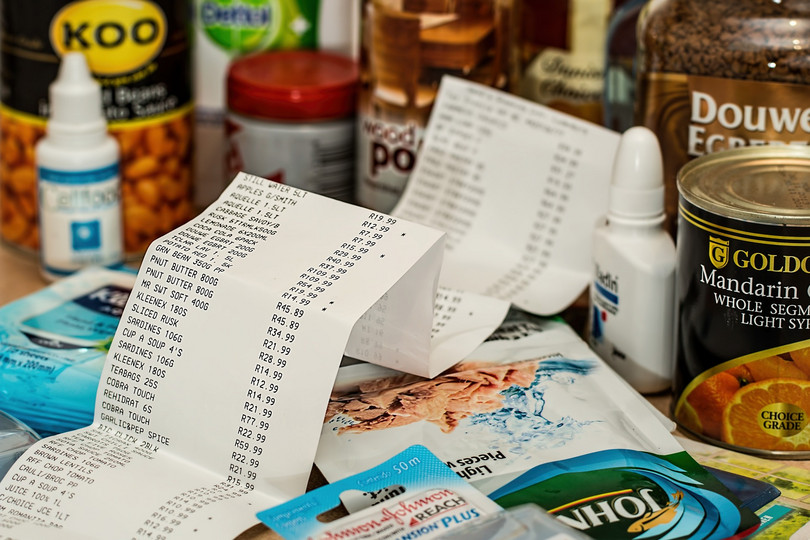Home Practice
For learners and parents For teachers and schools
Textbooks
Full catalogue
Pricing SupportLog in

We think you are located in United States. Is this correct?

# Test yourself now

High marks in science are the key to your success and future plans. Test yourself and learn more on Siyavula Practice.Working with money involves decimal numbers because of the way our currency works. If an item costs $$25$$ rand and $$99$$ cents, the price is written as a decimal number: $$\text{R}\,\text{25,99}$$. If another item costs $$\text{R} \text{120,50}$$ and we want to buy both items, then we need to be able to add the two decimal numbers to find the total. If we want to check the change from $$\text{R}\,200$$, we will also need to know how to subtract decimal numbers.